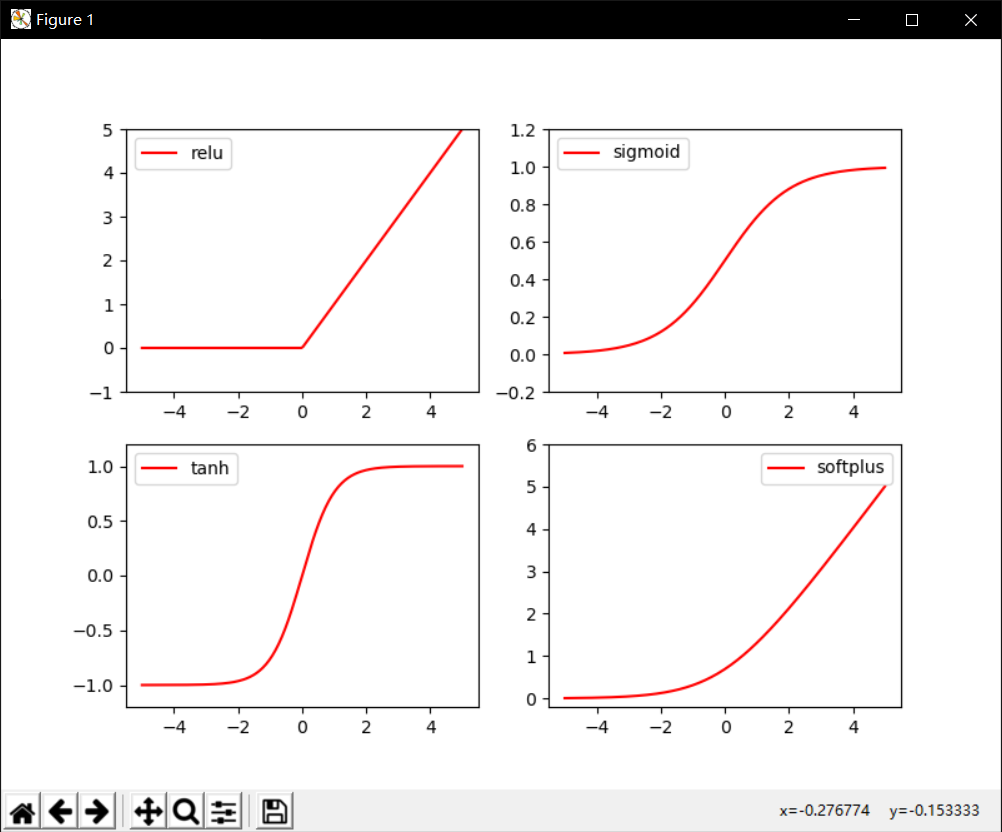+关注

2019-08(85)

2019-09(108)

2019-10(13)

2019-11(11)

2019-12(17)

## Pytorch中的激活函数

``````在某种意义上讲，深度学习=神经网络

x0 -> y_1=f(Wx0) -> y_2=f(Wx1) -> y_3=f(Wx2) -> . . . -> y=f(Wx(n-1))

先进行一轮“直”（线性的）的操作——y。=Wx，就是个加权，y。中的每一个数据都是对x中所有数据的一个加权和
再进行一轮“弯”（非线性的）的操作——y_ =f(y。)，其中f()是一个非线性的函数，它把直的掰弯，可以构造更多的可能，拓展了深度学习的应用领域
*注：完整的“直”（线性的）操作部分应该还有一个偏置b，即y。= Wx+b，这里为了简化说明，就默认b=0了
```1234567```

Pytorch是一个机器学习库，封装了大量机器学习的方法，这里说一下其中的激活函数，就是上面说的 f()

• 导入支持包

``````import torch
import torch.nn.functional as F     # 激励函数都在这
```123```
• 生成输入数据

``````# 做一些假数据来观看图像
x = torch.linspace(-5,5,200).unsqueeze(1)   # .unsqueeze()作用为增加1个维度，x.size=(200,1)
x = torch.tensor(x)  # x data (tensor), shape=(100, 1)
x = Variable(x)
```1234```
• “直”（线性）的操作

激活函数可以理解为 y_=f(Wx) ,

为了后面更好的观察激活函数的效果， 这里令W=“1”， 即y=x，则y_=f(Wx)=f(x)

整个网络假设就这么一层

``````W = torch.eye(200)   # 对角矩阵
# 当传入值为5是，W=
# tensor([[1., 0., 0., 0., 0.],
#         [0., 1., 0., 0., 0.],
#         [0., 0., 1., 0., 0.],
#         [0., 0., 0., 1., 0.],
#         [0., 0., 0., 0., 1.]])
W = Variable(W)
```12345678```
• y_ = Wx

``````y_ = W.mm(x)     # Wx, mm()是pytorch中的矩阵点乘
```1```
• 激活

``````# 几种常用的 激励函数    y_activate=f(y)=f(Wx)
y_relu = F.relu(y_)
y_sigmoid = F.sigmoid(y_)
y_tanh = F.tanh(y_)
y_softplus = F.softplus(y_)
# y_softmax = F.softmax(y)  softmax 比较特殊, 不能直接显示, 不过他是关于概率的, 用于分类
```123456```
• 作图观察

``````# 作图观察
import matplotlib.pyplot as plt  # python 的可视化模块

plt.figure(1, figsize=(8, 6))
plt.subplot(221)
plt.plot(x, y_relu, c='red', label='relu')
plt.ylim((-1, 5))
plt.legend(loc='best')

plt.subplot(222)
plt.plot(x, y_sigmoid, c='red', label='sigmoid')
plt.ylim((-0.2, 1.2))
plt.legend(loc='best')

plt.subplot(223)
plt.plot(x, y_tanh, c='red', label='tanh')
plt.ylim((-1.2, 1.2))
plt.legend(loc='best')

plt.subplot(224)
plt.plot(x, y_softplus, c='red', label='softplus')
plt.ylim((-0.2, 6))
plt.legend(loc='best')

plt.show()
```12345678910111213141516171819202122232425```• 说明

程序主要来自 激励函数 (Activation)，略有修改

将程序按顺序复制即可执行

• 参考文献

激励函数 (Activation)

3 0